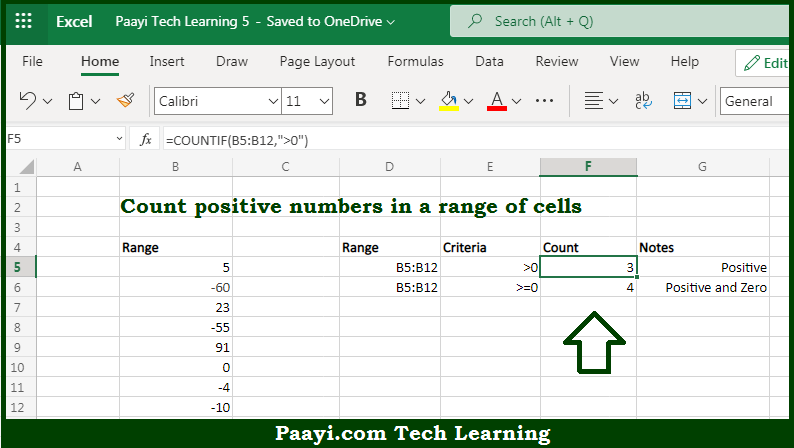# Learn How to Count Cells that Contain Positive Numbers in Microsoft Excel

Written by | 0 Comments | 489 Views

In this article, you will learn how to COUNT various things in Microsoft Excel using a single or combination of functions and its purpose in Microsoft Excel. You will also get to know how to count cells that contain positive numbers & generic formula

Count Cells that Contain Positive Numbers in Microsoft Excel

The main purpose of this formula is to count the number of cells that contain positive numbers. Here we will learn how to count the range of cells containing the positive numeric values in Microsoft Excel.  That implies, with the help of a formula based on the  COUNTIF function you can able to count the number of cells that contain only positive numbers. The generic form of the formula provided below "rng" represents a range of cells. So, with the help of this formula, you can able to count the number of cells that contain positive numbers.

General Formula to COUNT Cells that Contain Positive Numbers

=COUNTIF(rng,">0")

The Explanation for the COUNT Cells that Contain Positive NumbersSo we know that with the help of the given formula above you can able to count the number of cells that contain positive numbers. Here we will learn how to count the range of cells containing the positive numeric values in Microsoft Excel. As we know that, the  COUNTIF function is used to count the number of cells in a range that matches the supplied criteria. In the example given here, the criteria are supplied as ">0", which is evaluated as "values greater than zero". It should be noted that the COUNTIF function returns the count of all cells in the range that meet these criteria. So, with the help of this formula, you can able to count cells that contain positive numbers.